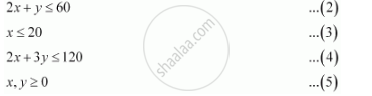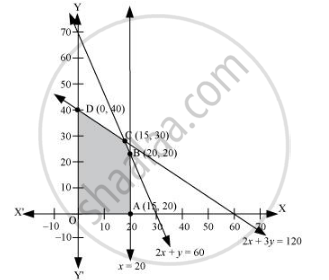Share

# A Manufacturer Makes Two Types of Toys a and B. Three Machines Are Needed for this Purpose and the Time (In Minutes) Required for Each Toy on the Machines is Given Below Show that 15 Toys of Type a and 30 of Type B Should Be Manufactured in a Day to Get Maximum Profit. - CBSE (Science) Class 12 - Mathematics

ConceptLinear Programming Problem and Its Mathematical Formulation

#### Question

A manufacturer makes two types of toys A and B. Three machines are needed for this purpose and the time (in minutes) required for each toy on the machines is given below:

 Type of toy Machines I II III A 12 18 6 B 6 0 9

Each machine is available for a maximum of 6 hours per day. If the profit on each toy of type A is Rs 7.50 and that on each toy of type B is Rs 5, show that 15 toys of type A and 30 of type B should be manufactured in a day to get maximum profit.

#### Solution

Let x and y toys of type A and type B respectively be manufactured in a day.

The given problem can be formulated as follows.

Maximize z = 7.5x + 5y … (1)

subject to the constraints,The feasible region determined by the constraints is as follows.The corner points of the feasible region are A (20, 0), B (20, 20), C (15, 30), and D (0, 40).

The values of z at these corner points are as follows.

 Corner point Z = 7.5x + 5y A(20, 0) 150 B(20, 20) 250 C(15, 30) 262.5 → Maximum O(0, 40) 200

The maximum value of z is 262.5 at (15, 30).

Thus, the manufacturer should manufacture 15 toys of type A and 30 toys of type B to maximize the profit.

Is there an error in this question or solution?

#### APPEARS IN

Solution A Manufacturer Makes Two Types of Toys a and B. Three Machines Are Needed for this Purpose and the Time (In Minutes) Required for Each Toy on the Machines is Given Below Show that 15 Toys of Type a and 30 of Type B Should Be Manufactured in a Day to Get Maximum Profit. Concept: Linear Programming Problem and Its Mathematical Formulation.
S